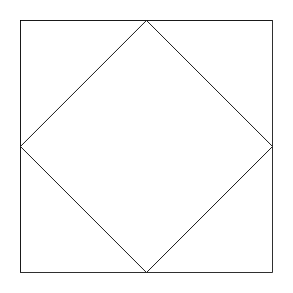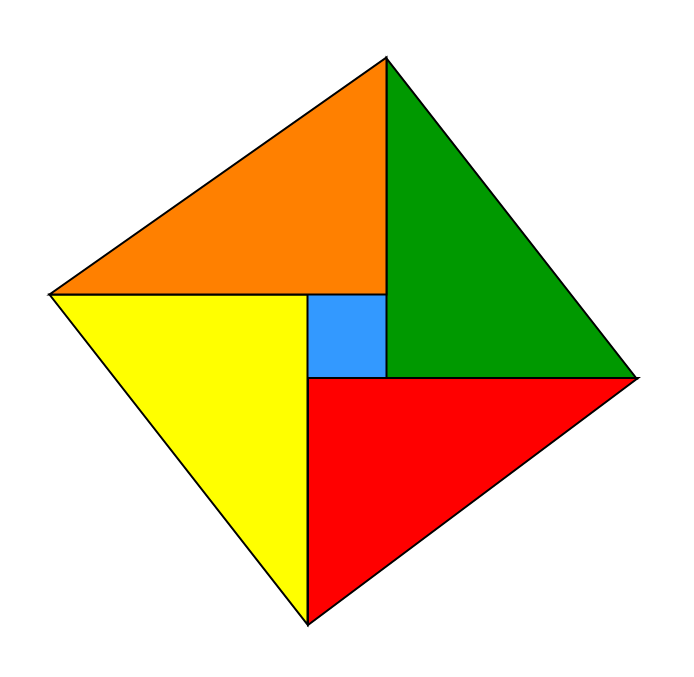# Puzzle | (Square and Right angles, Up the Ladder)

• Difficulty Level : Easy
• Last Updated : 07 Feb, 2018

Puzzle 1 : Square and Right angles

Can you make 2 squares and 4 right angled triangles using only 8 straight lines ?
Solution: This is the simple solution for the above problem. Here we use 8 straight lines to make 2 squares and 4 right angle triangles.Puzzle 2 : Up the Ladder

Suppose you want to reach up a window 40 feet from the ground. The distance from the foot of the ladder to the wall is 9 feet. Then, according to you, how long should the ladder be ?
Solution: We can easily solve this problem by using Pythagoras theorem. Consider the length of the ladder be X,
then
x^2 = 40^2 + 9^2
x^2 = 1681
x = 41 feet

The ladder should be 41 feet long.

This article is contributed by Bishal Kumar Dubey. If you like GeeksforGeeks and would like to contribute, you can also write an article using contribute.geeksforgeeks.org or mail your article to contribute@geeksforgeeks.org. See your article appearing on the GeeksforGeeks main page and help other Geeks.